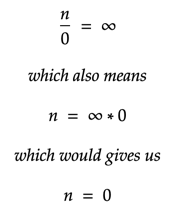# Why isn’t n/0 allowed in mathematics.

If you divide 4 by 2 you would get 2 as the quotient, which means there are exactly two 2’s in 4. And similarly, If you divide 5 by 2, you would get 2 as the quotient and 1as the remainder, which means there are two 2’s in 5 and a 1 which if you want can be further divided into 0.5 and 0.5 giving us 2.5 as the final result of the division.

Similarly, if you were to divide any number n by 0, there aren’t any 0’s you can take out of n. If you could, then you might think you can take out an infinite number of 0’s? This represented in mathematical form gives us,From our previous example, we can say, 5/2 = 2.5 or 2.5*2 = 5 but we can’t say n/0 = x or x*0 = n because multiplying any number with 0 is supposed to be a 0. So 1/0, 2/0, 3/0 .. etc are all meaningless in mathematics. And, If division by zero was allowed then we can deduce absurd relations like 1=2 from 1*0 = 2*0 (allowing 0/0).